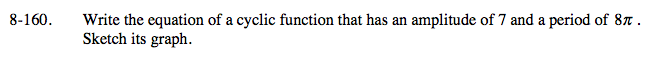### Home > A2C > Chapter 8 > Lesson 8.2.4 > Problem8-160

8-160.In the general equation y = a · sin[b(xh)] + k, the parameter a is the amplitude and b is the number of cycles in 2π.

If you need help, see the Math Notes box for this lesson and problem 8-159.

Test your equation on a graphing calculator to see if it meets the given criteria.